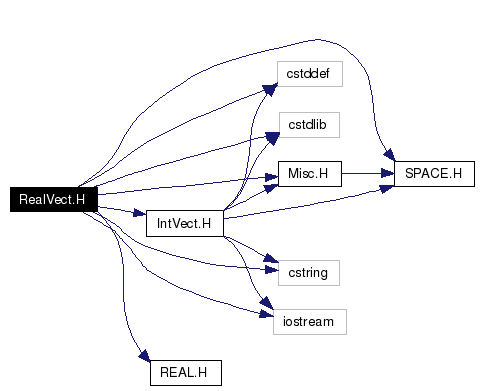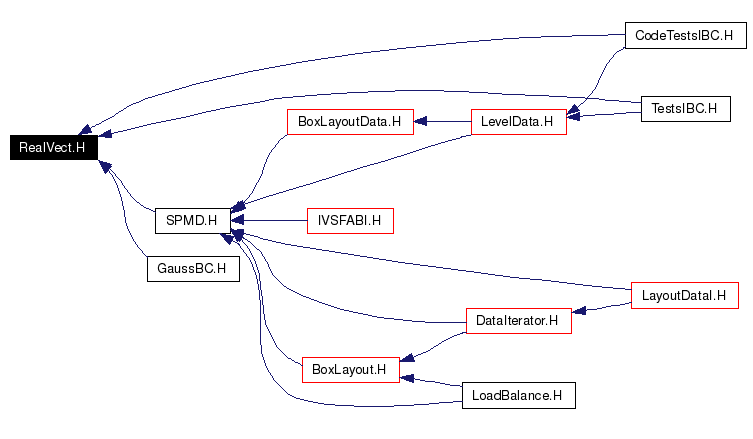# RealVect.H File Reference

`#include <cstddef>`
`#include <cstdlib>`
`#include <cstring>`
`#include <iostream>`
`#include "SPACE.H"`
`#include "Misc.H"`
`#include "REAL.H"`
`#include "IntVect.H"`

Include dependency graph for RealVect.H:This graph shows which files directly or indirectly include this file:Go to the source code of this file.

## Defines

#define LLT0   (vect < s)
#define LLT1   ((vect == s) && (vect < s))
#define LLT2   ((vect == s) && (vect < s))
#define LGT0   (vect > s)
#define LGT1   ((vect == s) && (vect > s))
#define LGT2   ((vect == s) && (vect > s))

## Functions

RealVect scale (const RealVect &p, Real s)
RealVect BASISREALV (int idir)

## Define Documentation

 #define LGT0   (vect > s)

 #define LGT1   ((vect == s) && (vect > s))

 #define LGT2   ((vect == s) && (vect > s))

 #define LLT0   (vect < s)

 #define LLT1   ((vect == s) && (vect < s))

 #define LLT2   ((vect == s) && (vect < s))

## Function Documentation

 RealVect BASISREALV ( int idir )
 Returns a basis vector in the given coordinate direction. In 2-D: BASISREALV(0) == (1.,0.); BASISREALV(1) == (0.,1.). In 3-D: BASISREALV(0) == (1.,0.,0.); BASISREALV(1) == (0.,1.,0.); BASISREALV(2) == (0.,0.,1.). Note that the coordinate directions are based at zero.

 RealVect scale ( const RealVect & p, Real s ) ` [inline]`
 Returns a RealVect obtained by multiplying each of the components of the given RealVect by a scalar.

Generated on Wed Oct 5 13:58:19 2005 for Chombo&AMRSelfGravity by1.4.1